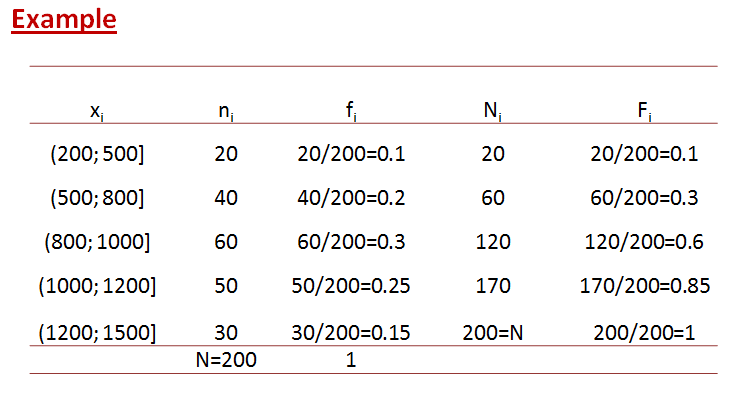# Frequency distributions

Frequency distributions are used to organize the information of the variables. Considering the nature of the variable they could be organized in groups or without groups.

## Ungrouped data

Absolute frequency ($n_{i}$): Number of times that a value is repeated in the sample.

Relative frequency ($f_{i}$): Absolute frequency divided by the total number of observations. It represents the percentage of times that the value is repeated in the sample.

Accumulated absolute frequency ($N_{i}$): Number of observations smaller or equal than the considered value.

Accumulated relative frequency ($F_{i}$): Percentage of observations smaller or equal than the considered value. It is calculated by dividing the accumulated absolute frequencies by the sample size.• Relative frequencies sum up to one.
• The last accumulated relative frequency is unity.

## Grouped data

When the variability of the variable is short, like in the example, it is not necessary create groups for values. If the variability is larger it becomes necessary organize the information in groups.

The maximum information we have by collecting the data, since after grouping we lose information.

It is important consider that the intervals are ad-hoc, i.e., they are determined by the researcher and not by the distribution of the data. The intervals have the following characteristics:$L_{i}$: Upper limit of the interval.$L_{i-1}$: Lower limit of the interval.
Range of the variable:$\boxed{Re=\max_{i}x_{i}-\min_{i}x_{i}}$
Length of the interval:$\boxed{c_{i}=L_{i}-L_{i-1}}$

The length can be constant (easier to deal with) or variable. If it is constant, we can find the number of intervals by fixing their length or the length by fixing the number of intervals:There is no rule of thumb regarding the number of intervals, it is usually between 5 and 15.

The intervals, as a general rule, are left-open right-closed intervals, i.e.$\left(a,b\right]$.

Midpoint ($x_{i}$): It is the point in the middle of each interval. It represents the interval. It is calculated by:$x_{i}=\frac{L_{i-1}+L_{i}}{2}$